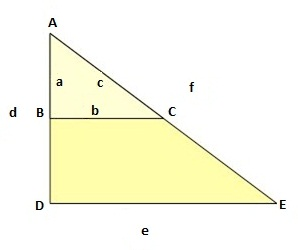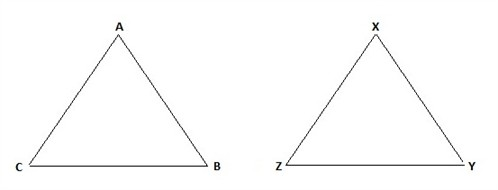# Similar figures

We often come across two triangles that share the same proportions, i.e. the angles are alike though their sizes differ. These are called similar triangles. The simplest method of illustrating two similar triangles is first to draw a triangle, and then to draw a straight line so that we now has a smaller triangle within the large one.Triangles that are similar are shown as

$\bigtriangleup ABC\sim \bigtriangleup DEF$

The sides that have the same relative position in the similar figures, like A and D in the triangle above are called corresponding sides. The ratios of the corresponding sides of similar figures are equal which means that they are proportions.

$\frac{a}{d}=\frac{b}{e}=\frac{c}{f}\: \: or\:\: \frac{d}{a}=\frac{e}{b}=\frac{f}{c}$

Figures that have both the same size and shape are called congruent figures and are indicated as

$\bigtriangleup ABC\cong \bigtriangleup XYZ$